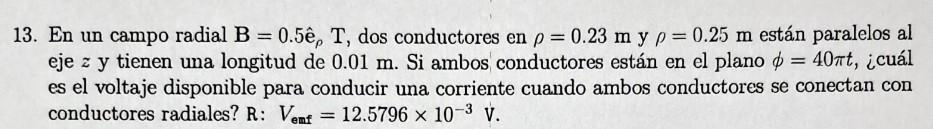# (Solved): 13. In a radial field B = 0.5, T, two conductors at p = 0.23 m and p=0.25 m are parallel to the z ...

13. In a radial field B = 0.5ê, T, two conductors at p = 0.23 m and p=0.25 m are parallel to the z axis and have a length of 0.01 m. If both conductors are in plane = 40πt, what is the voltage available to drive a current when both conductors are connected to radial conductors? A: Vent = 12.5796 x 10-3V.3. En un campo radial , dos conductores en y están paralelos al eje y tienen una longitud de . Si ambos conductores están en el plano , ¿cuál es el voltaje disponible para conducir una corriente cuando ambos conductores se conectan con conductores radiales? R: .

We have an Answer from Expert

La tensión disponible para impulsar una corriente cuando ambos conductores están conectados a conductores radiales se puede determinar utilizando la ley de inducción electromagnética de Faraday. Según esta ley, la fuerza electromotriz inducida (o voltaje) en un circuito cerrado es igual a la tasa de cambio negativa del flujo magnético a través del circuito.

Suponiendo que el campo magnético es constante y que los conductores se mueven en el campo radial, el cambio en el flujo magnético a través del circuito formado por los conductores se debe al cambio en el área del circuito. Esta área está cambiando porque la distancia radial de los conductores está cambiando con el tiempo.

El cambio en el área del circuito se puede calcular como la diferencia en las distancias radiales de los conductores   multiplicado por la longitud de los conductores    lo que da
We have an Answer from Expert